# Inter maths IIA solutions for quadratic expressions exercise 3(c)

Intermediate second year mathematics quadratic expressions exercise 3(c) solutions ( for text book problems) are given below.

These solutions are very easy to understand.

You can also see the solurions for first year maths 1A and 1B for examination purpose.

Inter maths 1A solutions

3. Matrices

You can also see the solutions for 1b

1. Locus

The straight line sa

Straight lines la

12. Rate measure

You can see the solutions for maths IIA inter

Complex numbers solutions for textbook

De Moivre’s Theorem textbook solutions

Exercise 3(b)

Exercise 3(c)

You can also see solutions for Inter maths IIB

1. Circle

3. Parabola

4. Ellipse

# Quadratic expressions exercise 3(c) inter

Exercise 3(c)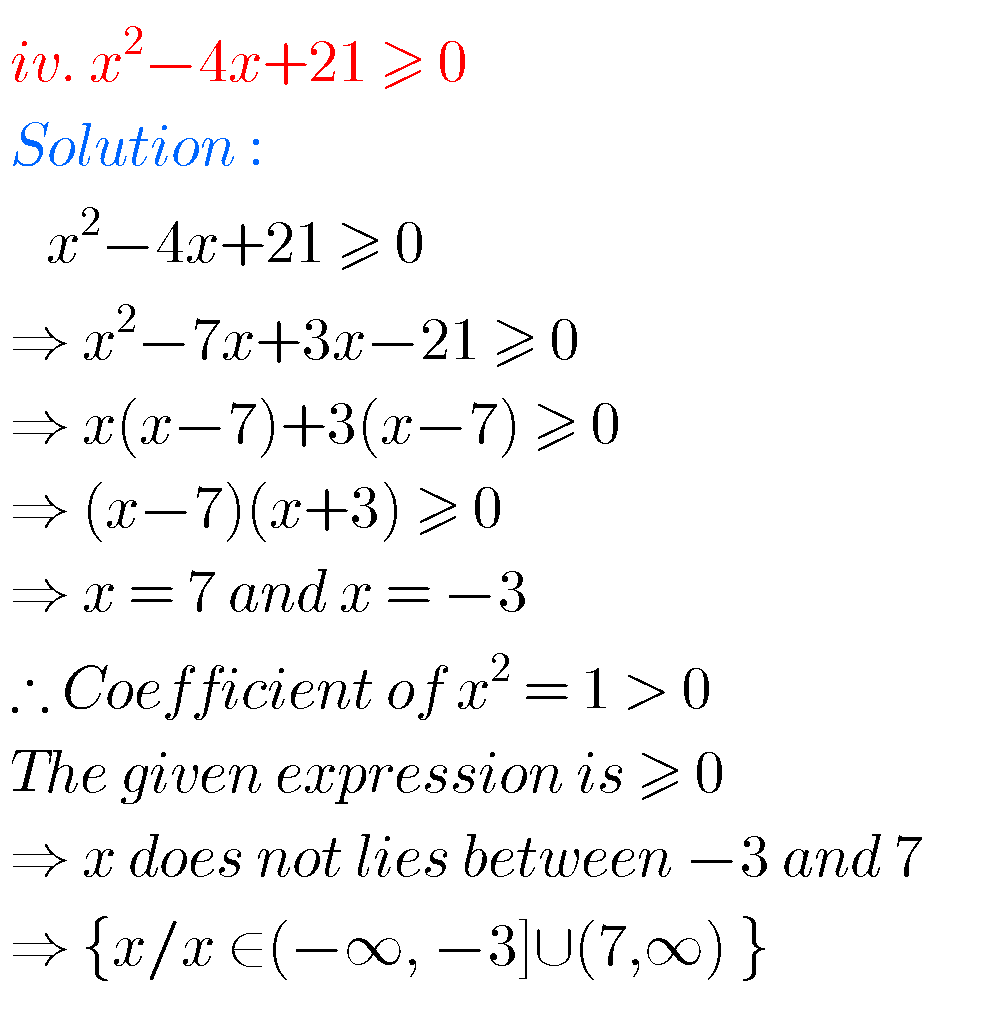GraphGraph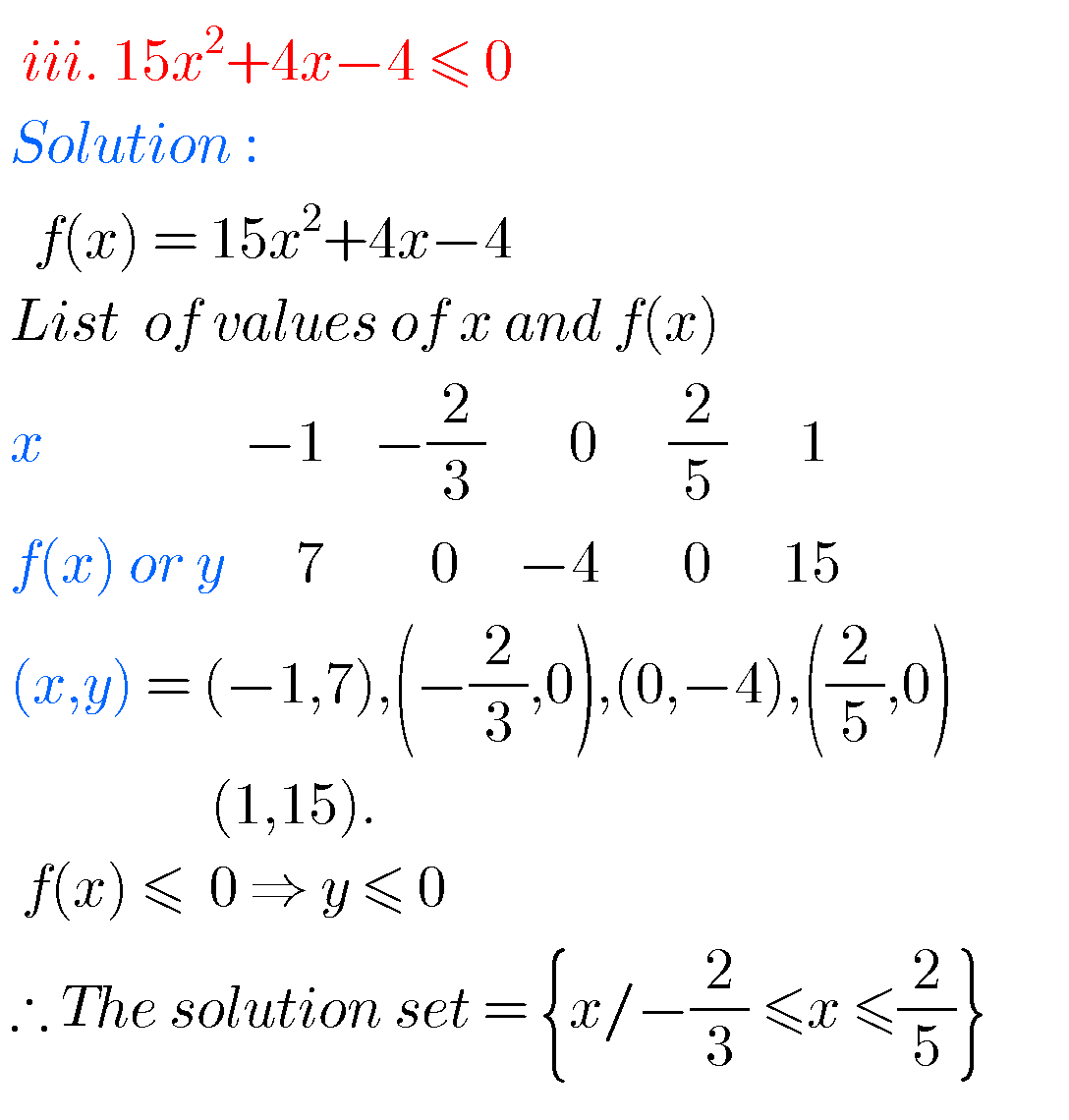Graph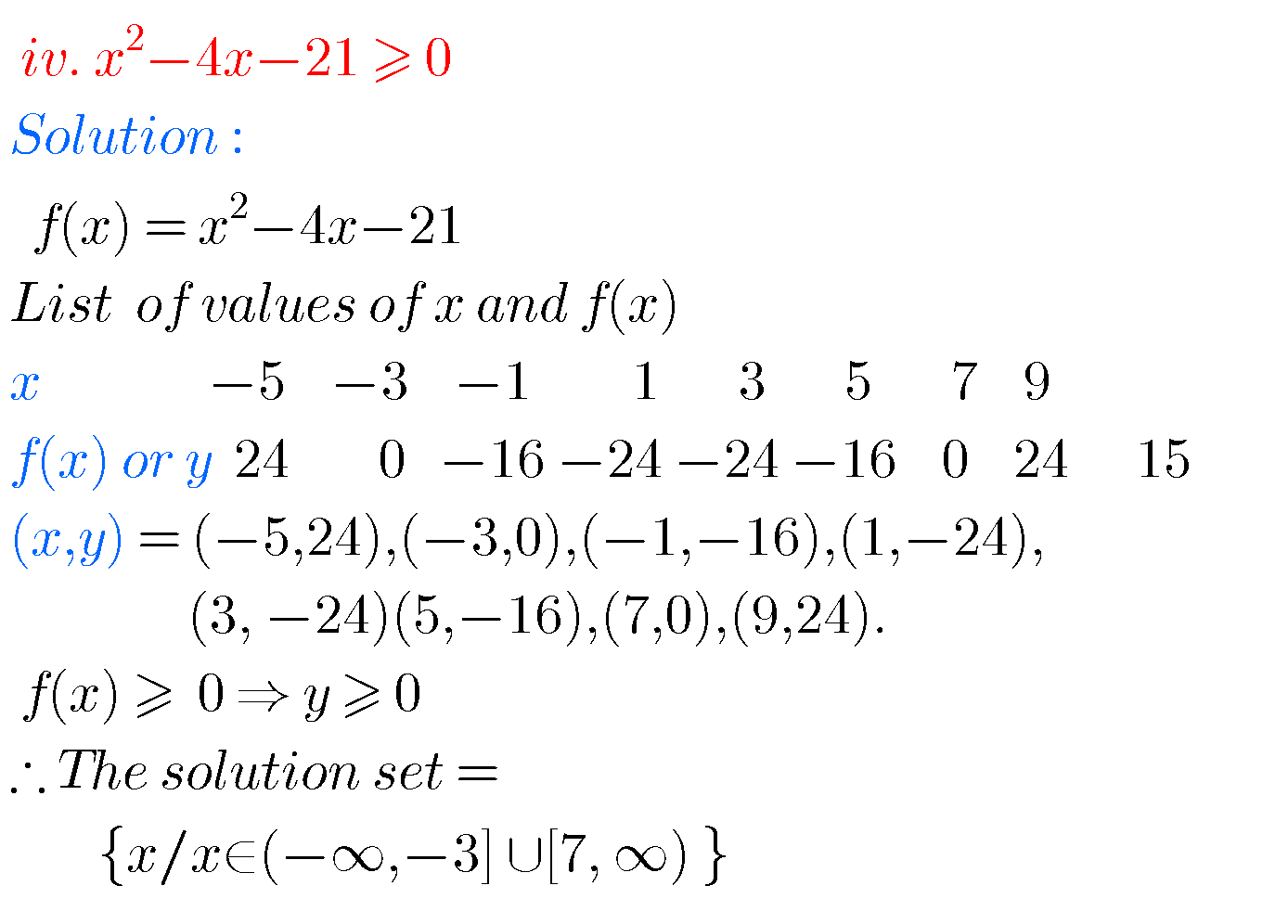Graph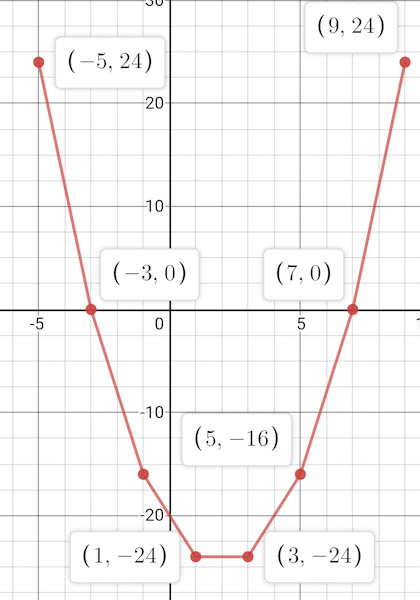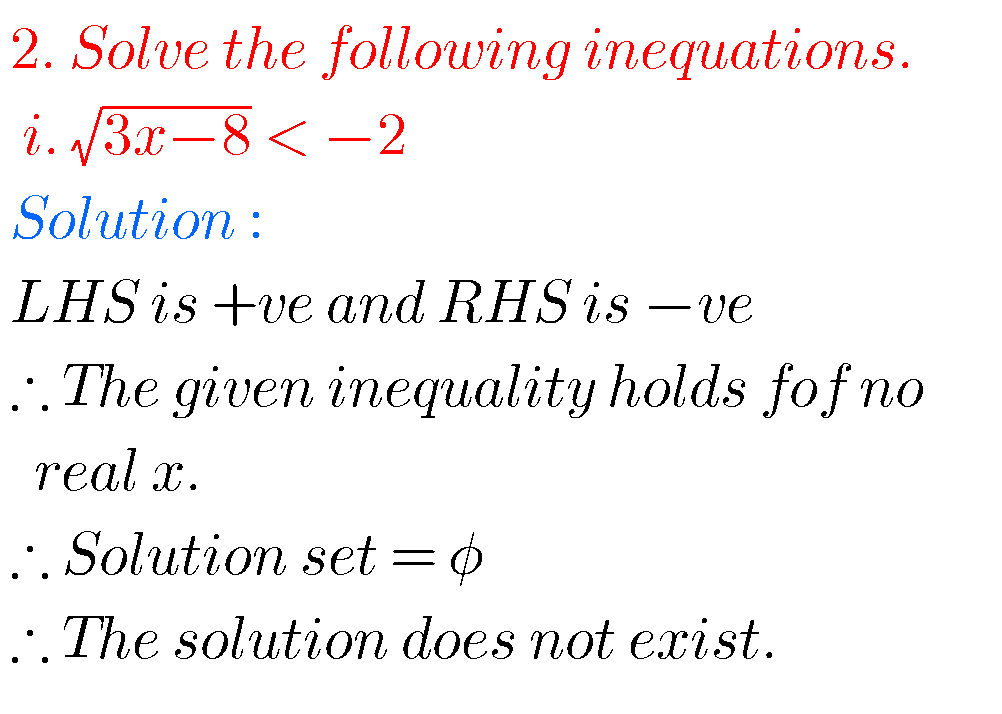Note : Observe the solutions and try them in your own methods.

class 10 maths polynomials solutions

Ncert maths class 8 exponents and powers

Ncert maths class 7 fractions and decimals

Ncert maths class 6 playing with numbers